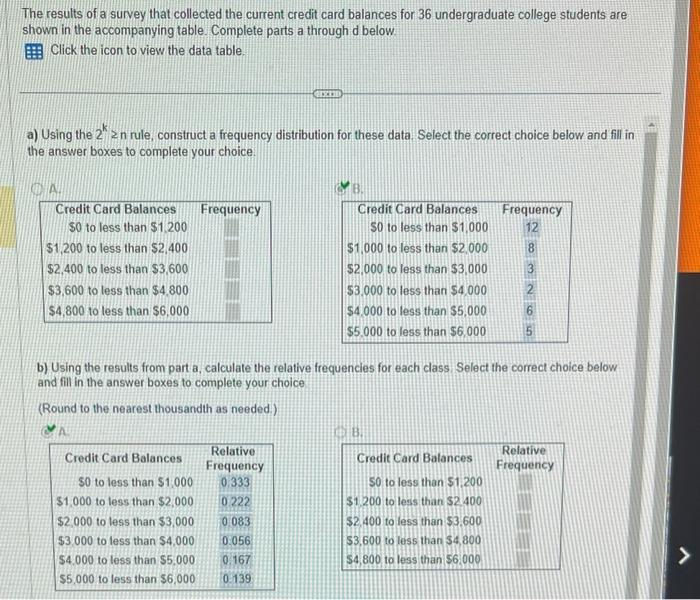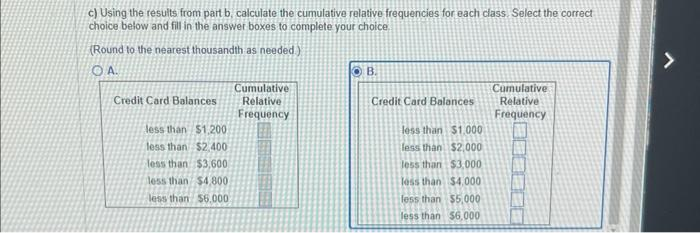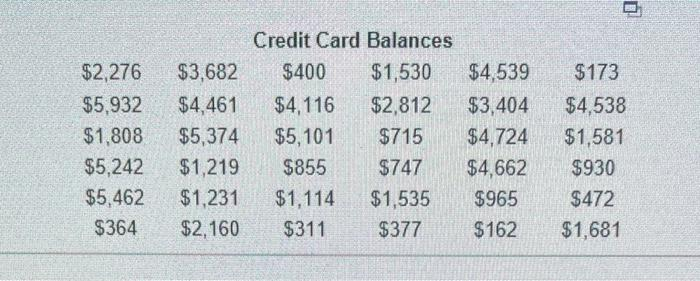# (Solved): The results of a survey that collected the current credit card balances for 36 undergraduate colleg ...The results of a survey that collected the current credit card balances for 36 undergraduate college students are shown in the accompanying table. Complete parts a through $$d$$ below. Click the icon to view the data table. a) Using the $$2^{k} \geq$$ n rule, construct a frequency distribution for these data. Select the correct choice below and fill in the answer boxes to complete your choice. b) Using the results from part a, calculate the relative frequencies for each class Select the correct choice below. and fill in the answer boxes to complete your choice (Round to the nearest thousandth as needed) c) Using the results from part b, calculate the cumulative relative frequencies for each class. Select the correct choice below and fill in the answer boxes to complete your choice. (Round to the nearest thousandth as needed) \begin{tabular}{cccccc} \multicolumn{5}{c}{ Credit Card Balances } \\ $$\ 2,276$$ & $$\ 3,682$$ & $$\ 400$$ & $$\ 1,530$$ & $$\ 4,539$$ & $$\ 173$$ \\ $$\ 5,932$$ & $$\ 4,461$$ & $$\ 4,116$$ & $$\ 2,812$$ & $$\ 3,404$$ & $$\ 4,538$$ \\ $$\ 1,808$$ & $$\ 5,374$$ & $$\ 5,101$$ & $$\ 715$$ & $$\ 4,724$$ & $$\ 1,581$$ \\ $$\ 5,242$$ & $$\ 1,219$$ & $$\ 855$$ & $$\ 747$$ & $$\ 4,662$$ & $$\ 930$$ \\ $$\ 5,462$$ & $$\ 1,231$$ & $$\ 1,114$$ & $$\ 1,535$$ & $$\ 965$$ & $$\ 472$$ \\ $$\ 364$$ & $$\ 2,160$$ & $$\ 311$$ & $$\ 377$$ & $$\ 162$$ & $$\ 1,681$$ \end{tabular}

We have an Answer from Expert